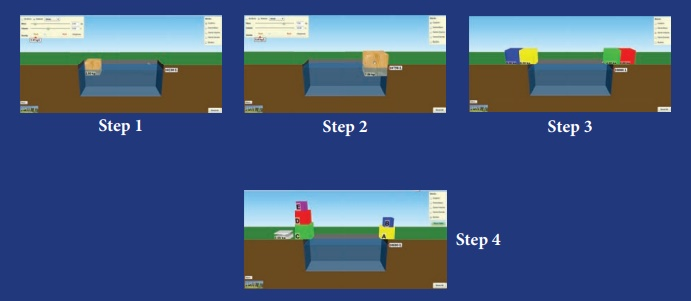Home | | Science 7th Std | Points to remember

# Points to remember

7th Science : Term 1 Unit 1 : Measurement : Points to remember

Points to remember:

* A set of physical quantities which cannot be expressed in terms of any other quantities are known as “Fundamental quantities”. Their corresponding units are called “Fundamental units”.

* The physical quantities which can be obtained by mathematically combining (i.e., multiplying and dividing) the fundamental quantities are known as “Derived quantities”. Their corresponding units are called “Derived units”.

* The area of a figure is the region covered by the boundary of the figure. Its SI unit is square metre or m2.

* The area of irregularly shaped figures can be calculated with the help of a graph sheet.

* The amount of space occupied by a three dimensional object is known as its volume. The SI unit of volume is cubic metre or m3.

* The volume of liquids are expressed in terms of litre. One litre = 1000 cc.

* The maximum volume of a liquid that a container can bold is known as the capacity of the container.

* Density of a substance is defined as the mass of the substance contained in unit volume (1 m3).

* SI unit of density is kg/m3. The CGS unit of density is g/cm3. 1g/cm3 = 103 kg/m3.

* The materials with higher density are called “denser” and the materials with lower density are called “rarer”.

* If the density of a solid is higher than that of a liquid, it sinks in that liquid. If the density of a solid is lower than that of a liquid, it floats in that liquid.

* Density = Mass / Volume

* Mass = Density × Volume

* Volume = Mass / Density

* One astronomical unit is defined as the average distance between the earth and the sun. 1 AU = 149.6 × 106 km = 1.496 × 1011 m.

* One light year is defined as the distance travelled by light in vacuum during the period of one year. 1 Light year = 9.46 × 1015 m.

ICT CORNER

Measurement

Let’s know about the effects ofmass and volume on density.PROCEDURE :

Step 1: Use the URL or scan the QR code to open the activity page.

Step 2: Select the options at top right side window to customize

Step 3: Move the sliders on the top left-side window to change the Material and Mass, Volume. Now see the effects of mass and volume on density.

Step 4: Click ‘Reset all’ button to refreshMeasurement URL:

https://phet.colorado.edu/en/simulation/density (or) scan the QR Code

*Pictures are indicative only

*If browser requires, allow Flash Player or Java Script to load the page.

Tags : Measurement | Term 1 Unit 1 | 7th Science , 7th Science : Term 1 Unit 1 : Measurement
Study Material, Lecturing Notes, Assignment, Reference, Wiki description explanation, brief detail
7th Science : Term 1 Unit 1 : Measurement : Points to remember | Measurement | Term 1 Unit 1 | 7th Science